## Saturday, March 20, 2010

### Irodov Problem 3.17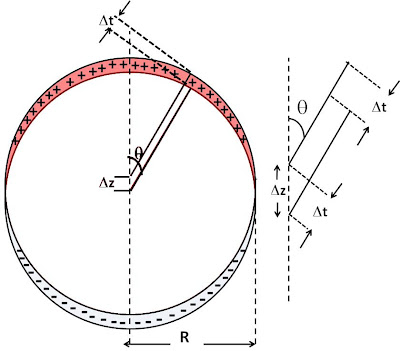a) First let us try and understand what happens in this problem. As shown in the figure, two charged spheres with charge densities areand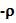respectively are placed on top of each other displaced by a small distance of. While the -ive and the +ive charges from the two spheres cancel in the center of the spheres. However, since the spheres are displaced with respect to each other (the upper sphere is +ively charged) around the periphery the charges will not cancel each other as shown in the figure. The amount of charge in the periphery will however, depend on the polar angle. As seen from the figure, the charge will be zero at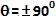and the maximum at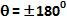. In fact what Irodov asks to prove is that is the displacementis small enough, then the two spheres can be replaced by a single hollow sphere with a surface change density that depends on the angleas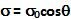.

To prove this, let us first determine the thickness of the charged section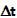as a function of the polar angle. As seen from the figure,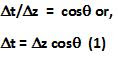Let us now consider an infinitesimally small piece of the charged part of the sphere that has the polar and azimuthal angles ofandrespectively and subtends a polar angle ofand an azimuthal angle ofas shown in the figure. The surface area of this section will be,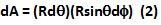The charge contained in this section will this be,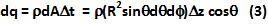If there were a single hollow sphere with a surface charge densitythen we would have,Comparing (3) and (4) we have,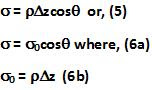b) Let us assume that the center of the positively charged sphere is located at the origin. The electric field inside the positively charged sphere distance r is given by,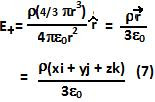Since the center of the negatively charged sphere is locatedunits below that of the positively charged sphere, the electric field due to this sphere is given by,The net electric field due to the two sphere are given by,